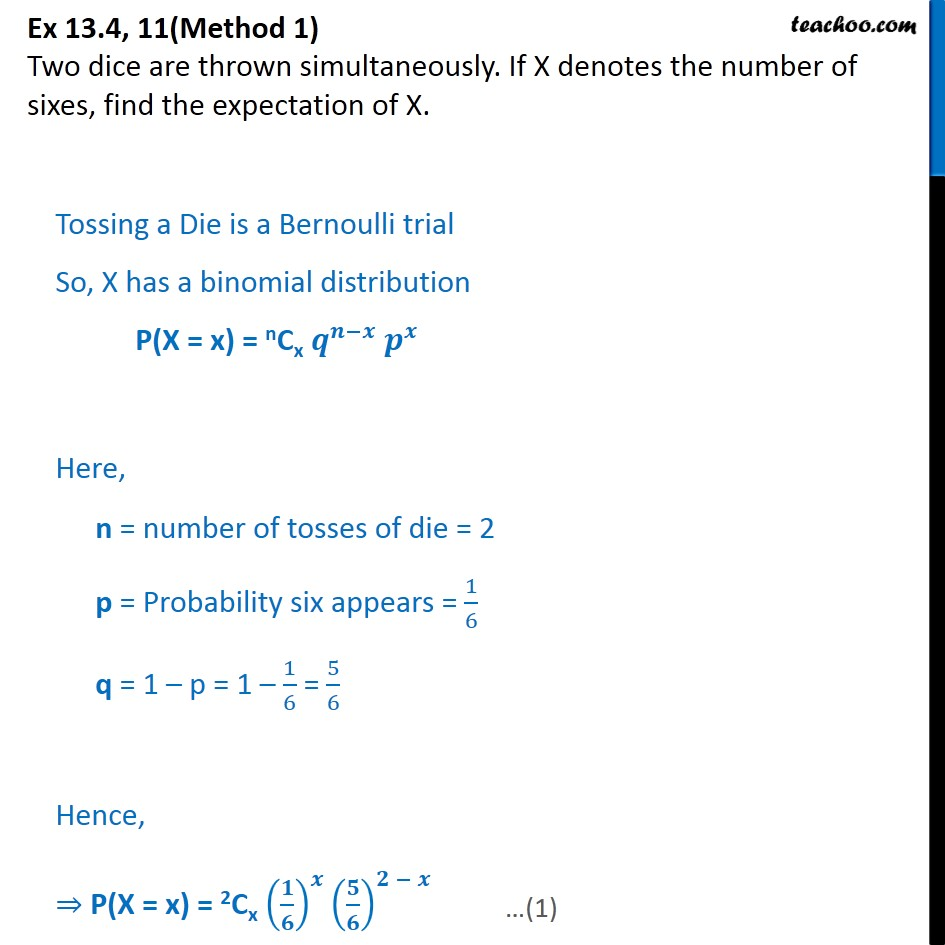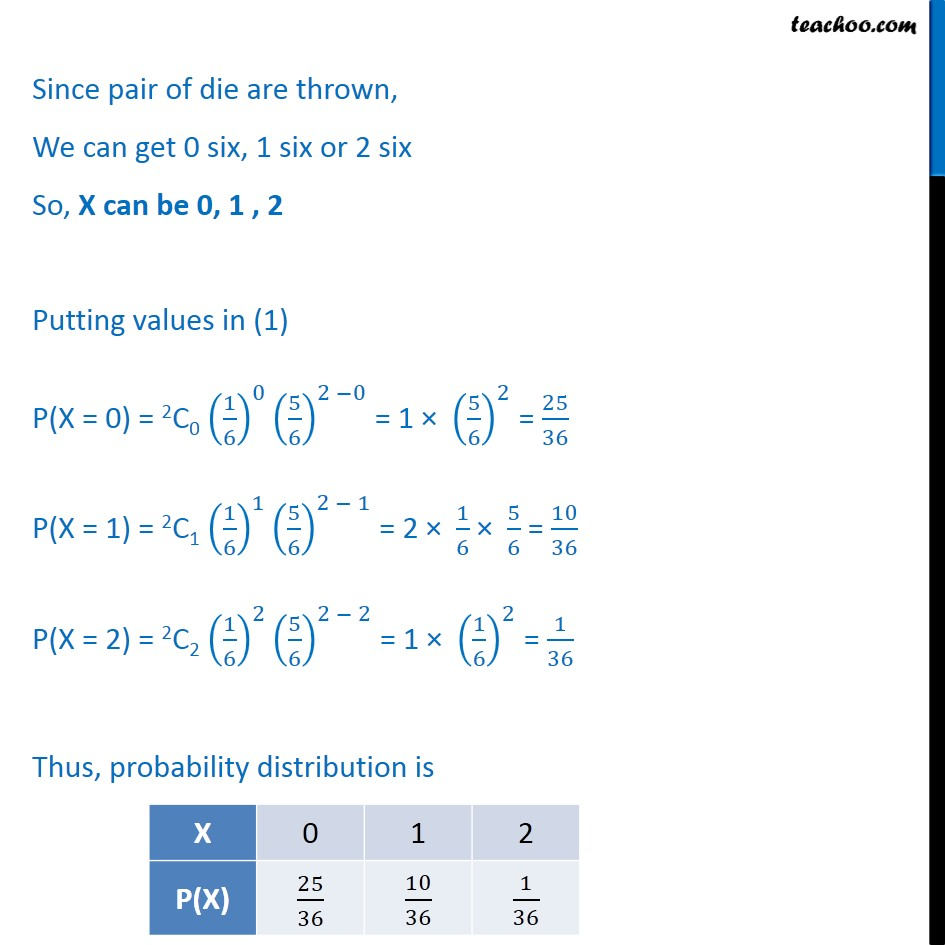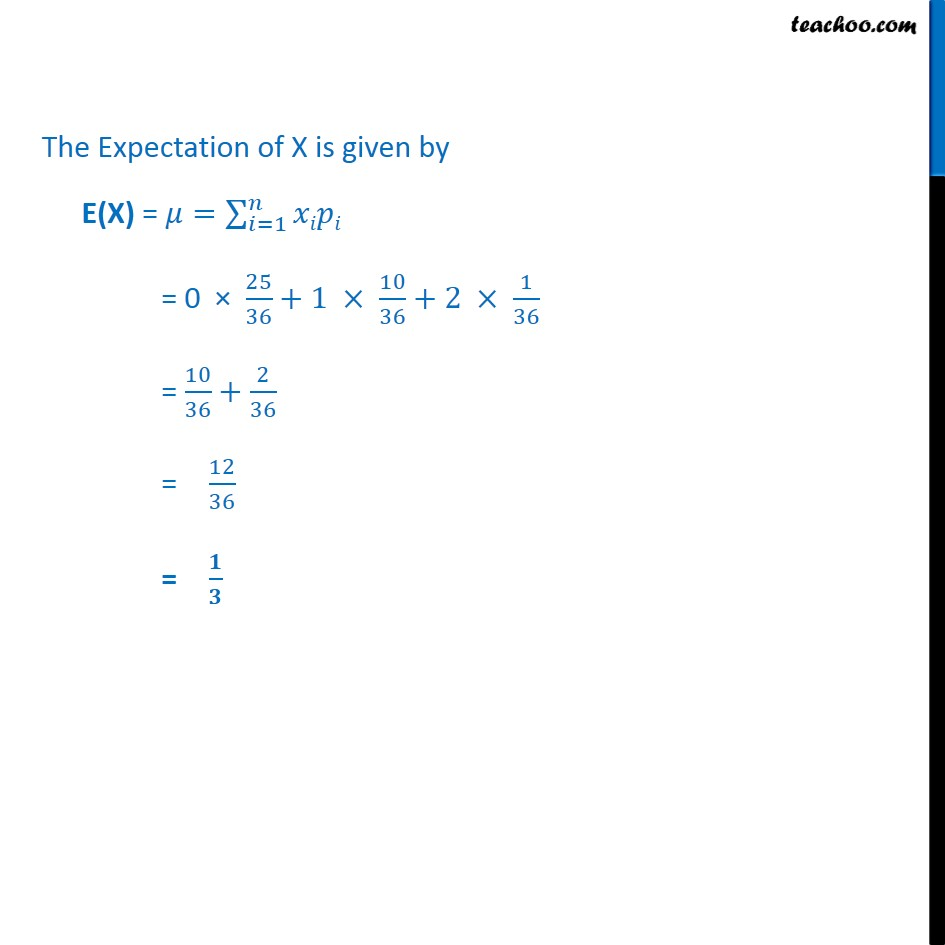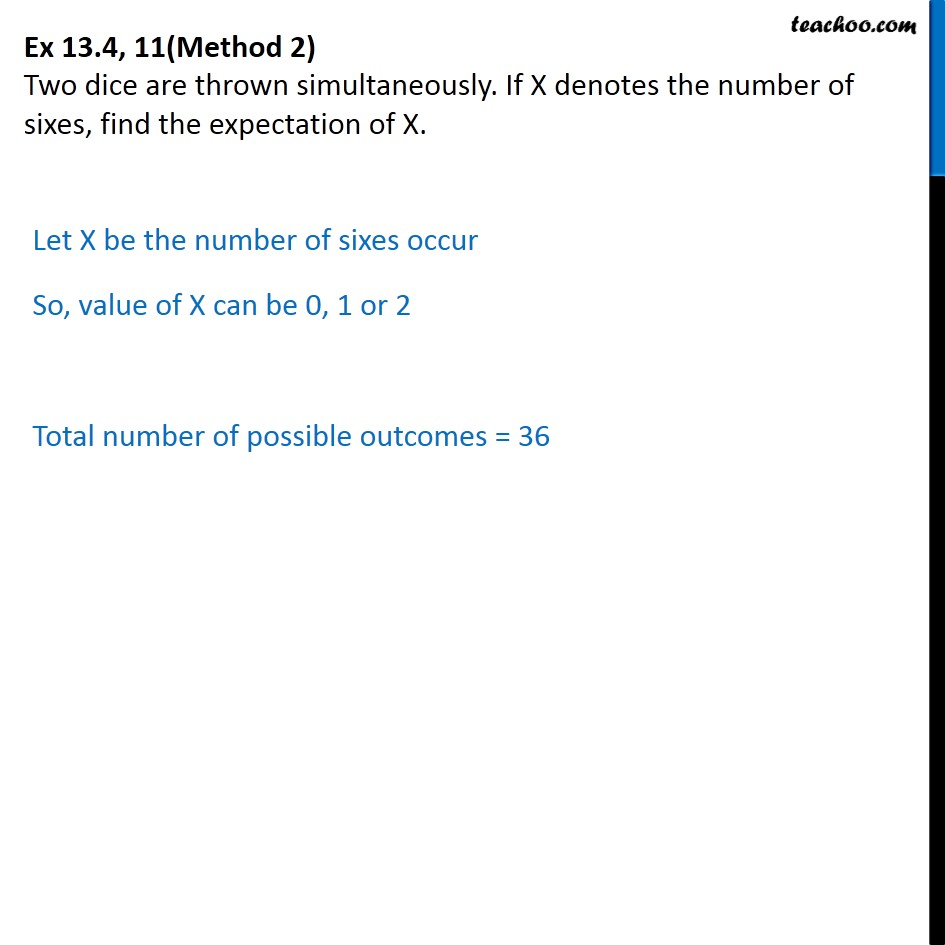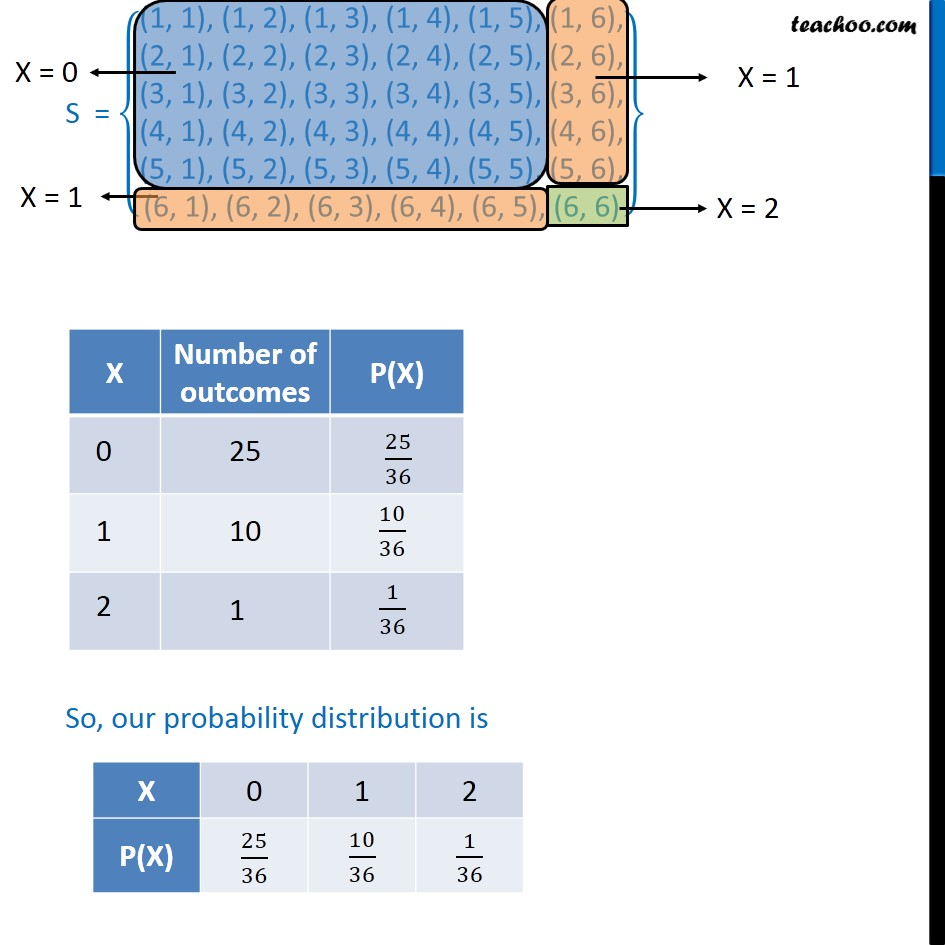1. Chapter 13 Class 12 Probability
2. Serial order wise
3. Ex 13.4

Transcript

Ex 13.4, 11(Method 1) Two dice are thrown simultaneously. If X denotes the number of sixes, find the expectation of X. Tossing a Die is a Bernoulli trial So, X has a binomial distribution P(X = x) = nCx 𝒒﷮𝒏−𝒙﷯ 𝒑﷮𝒙﷯ Here, n = number of tosses of die = 2 p = Probability six appears = 1﷮6﷯ q = 1 – p = 1 – 1﷮6﷯ = 5﷮6﷯ Hence, ⇒ P(X = x) = 2Cx 𝟏﷮𝟔﷯﷯﷮𝒙﷯ 𝟓﷮𝟔﷯﷯﷮𝟐 − 𝒙﷯ Since pair of die are thrown, We can get 0 six, 1 six or 2 six So, X can be 0, 1 , 2 Putting values in (1) P(X = 0) = 2C0 1﷮6﷯﷯﷮0﷯ 5﷮6﷯﷯﷮2 −0﷯ = 1 × 5﷮6﷯﷯﷮2﷯ = 25﷮36﷯ P(X = 1) = 2C1 1﷮6﷯﷯﷮1﷯ 5﷮6﷯﷯﷮2 − 1﷯ = 2 × 1﷮6﷯ × 5﷮6﷯ = 10﷮36﷯ P(X = 2) = 2C2 1﷮6﷯﷯﷮2﷯ 5﷮6﷯﷯﷮2 − 2﷯ = 1 × 1﷮6﷯﷯﷮2﷯ = 1﷮36﷯ Thus, probability distribution is The Expectation of X is given by E(X) = 𝜇= 𝑖=1﷮𝑛﷮𝑥𝑖𝑝𝑖﷯ = 0 × 25﷮36﷯+1 × 10﷮36﷯+2 × 1﷮36﷯ = 10﷮36﷯+ 2﷮36﷯ = 12﷮36﷯ = 𝟏﷮𝟑﷯ Ex 13.4, 11(Method 2) Two dice are thrown simultaneously. If X denotes the number of sixes, find the expectation of X. Let X be the number of sixes occur So, value of X can be 0, 1 or 2 Total number of possible outcomes = 36 S = (1, 1), (1, 2), (1, 3), (1, 4), (1, 5), (1, 6),﷮(2, 1), (2, 2), (2, 3), (2, 4), (2, 5), (2, 6),﷮(3, 1), (3, 2), (3, 3), (3, 4), (3, 5), (3, 6),﷮(4, 1), (4, 2), (4, 3), (4, 4), (4, 5), (4, 6),﷮(5, 1), (5, 2), (5, 3), (5, 4), (5, 5), (5, 6),﷮(6, 1), (6, 2), (6, 3), (6, 4), (6, 5), (6, 6)﷯﷯ So, our probability distribution is The Expectation of X is given by E(X) = 𝜇= 𝑖=1﷮𝑛﷮𝑥𝑖𝑝𝑖﷯ = 0 × 25﷮36﷯+1 × 10﷮36﷯+2 × 1﷮36﷯ = 10﷮36﷯+ 2﷮36﷯ = 12﷮36﷯ = 𝟏﷮𝟑﷯

Ex 13.4